# Proof I

When added to the product of two consecutive integers larger one, we get the square larger one. Is this true or not?

Result

### Step-by-step explanation:

$n\left(n+1\right)+\left(n+1\right)={n}^{2}+n+n+1={n}^{2}+2n+1=\left(n+1{\right)}^{2}$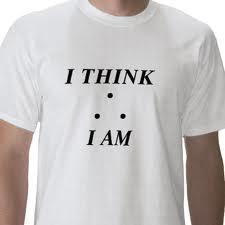Did you find an error or inaccuracy? Feel free to write us. Thank you!Tips to related online calculators
Do you have a linear equation or system of equations and looking for its solution? Or do you have a quadratic equation?

#### You need to know the following knowledge to solve this word math problem:

We encourage you to watch this tutorial video on this math problem:

## Related math problems and questions:

• Four integersFnd four consecutive integers so that the product of the first two is 70 times smaller than the product of the next two.
• Digits A, B, CFor the various digits A, B, C is true: the square root of the BC is equal to the A and sum B+C is equal to A. Calculate A + 2B + 3C. (BC is a two-digit number, not a product).
• Reciprocal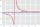It is true (prove it) that if a> b> 0: ?
• Proof PTCan you easily prove Pythagoras theorem using Euclidean theorems? If so, do it.
• ProductThe product of two consecutive odd numbers is 8463. What are this numbers?
• Two integers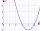Two integers, a and b, have a product of 36. What is the least possible sum of a and b?
• Three integersThree consecutive integers add up to 51. what are these integers?
• Powers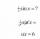Is true for any number a,b,c,d equality:? ?
• Larger perimeter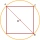There are a square and a circle that passes through two adjacent vertices of the square (end points of side a) and the center of the opposite side (c). Which of the plane shape has a larger perimeter?
• Hexagon - MOThe picture shows the ABCD square, the EFGD square and the HIJD rectangle. Points J and G lie on the side CD and is true |DJ|
• Three ints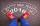The sum of three consecutive integers is 2016. What numbers are they?
• Square sides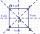If we enlarge the side of the square by a third, its circumference will be 20 cm larger than the original. What is the side of the square?
• Equilateral cylinder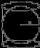A sphere is inserted into the rotating equilateral cylinder (touching the bases and the shell). Prove that the cylinder has both a volume and a surface half larger than an inscribed sphere.
• The sum 2The sum of five consecutive even integers is 150. Find the largest of the five integers. A.28 B.30 C.34 D.54 Show your solution and explain your answer.
• Rectangle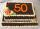Area of rectangle is 3002. Its length is 41 larger than the width. What are the dimensions of the rectangle?
• Product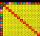The sum and the product of three integers are 6. Write largest of them.
• Find twoFind two consecutive natural numbers whose product is 1 larger than their sum. Searched numbers expressed by a fraction whose numerator is the difference between these numbers and the denominator is their sum.